Java语言之集合框架题白羽 2018-07-09 来源 ：网络 阅读 527 评论 0

问题：某班30个学生的学号为20070301-20070330,全部选修了Java程序设计课程，给出所有同学的成绩（可用随机数产生，范围60-100），请编写程序将本班各位同学的成绩按照从低到高排序打印输出。

要求：分别用List、Map、Set来实现，打印的信息包括学号、姓名和成绩。

```1、使用List集合来实现
[java] view plain copy
1. import java.util.ArrayList;
2. import java.util.Collections;
3. import java.util.Comparator;
4. import java.util.Iterator;
6. import java.util.TreeMap;
7.
8.
9.
10. public class Test2{
11.
12.     public static void main(String[] args){
13.
14.         /* 此处用ArrayList实现
15.          *
16.          * ArrayList<Student>al=new ArrayList<Student>();
17.         for(int i=20070301,j=10;i<=20070330;i++,j++)
18.         {
20.         }
21.
22.         //ArrayList排序借助Collections中的sort()方法实现。
24.         for(Student sd:al)
25.         System.out.println(sd);
26.
27.         */
28.
30.         for(int i=20070301,j=10;i<=20070330;i++,j++)
31.         {
33.         }
34.
35.         //对链表排序
37.         //输出链表
38.         for(Student sd:lt)
39.             System.out.println(sd);
40.
41.     }
42.
43. }
44.
45.
46.
47. //学生类
48. class Student{
50.     String name;
51.
52.     //构造函数
53.     public Student(int num,int grade,String name){
54.
55.         this.num=num;
56.         this.name=name;
58.     }
59.
60.     //此处必须覆写
61.     public String toString(){
62. //      System.out.println("hi");
64.     }
65.
66. }
67.
68. //创建一个比较器类
70.
71.     @Override
72.     public int compare(Student s1, Student s2) {
73.
75.             return 1;
77.             return -1;
79.             return s1.name.compareTo(s2.name);
80.         return 0;
81.
82.     }
83.
84. }

1、List集合其实是一个动态的数组，元素可以直接通过for循环取出，而不需要迭代。
2、输出List集合时，会默认调用集合中存储对象的toString（）方法，所以在类中需要进行覆写。

[java] view plain copy
1. for(int i=0;i<lt.size();i++)
2. {
3.     Student s=lt.get(i);
5. }

3、List集合的排序需要借助于Collections工具类，即Collections.Sort（list，new 比较器类（））方法。所以需要自定义一个比较器类，定义自己的比较规则。

2、使用Set集合来实现
（1）使用TreeSet来实现
[java] view plain copy
1. package com.package1;
2.
3. import java.util.*;
4.
5. public class StuScore {
6.
7. public static void main(String[] args) {
8.
9.     TreeSet<Student> ts=new TreeSet<Student>(new Com());
10.     //添加元素进去
11.     for(int i=20070301,j=1;i<=20070330;i++,j++)
12.     {
14.     }
15.
16.     //迭代循环取出
17.     Iterator<Student> it=ts.iterator();
18.     while(it.hasNext())
19.     {
20.         Student o1=it.next();
22.
23.     }
24.
25. }
26. }
27. //学生类
28. class Student
29. {
30. int num;
32. String name;
33.
34.
35. public Student(int num, String name,int grade)
36. {
37.     this.num=num;
38.     this.name=name;
40. }
41. }
42. class Com implements Comparator
43. {
44.
45. @Override
46. public int compare(Object o1, Object o2) {
47.
48.     Student s1=(Student) o1;
49.     Student s2=(Student) o2;
51.         return 1;
53.         return -1;
55.     {
56.         return new Integer(s1.num).compareTo(new Integer(s2.num));
57.     }
58.     return 0;
59. }
60. }

1、元素不可以重复，而且TreeSet是有序的。
2、两种排序方法：
（1）自定义一个比较器类，比如class Com implementsComparator{ }  ，实现compare(Object o1, Object o2)方法，在其中定义比较规则。
（2）让元素自身具备比较性。

用Comparator 的好处是不需要修改源代码, 而是另外实现一个比较器, 当某个自定义的对象需要作比较的时候,把比较器和对象一起传递过去就可以比大小了, 并且在Comparator 里面用户可以自己实现复杂的可以通用的逻辑,使其可以匹配一些比较简单的对象,那样就可以节省很多重复劳动了。

（2）使用HashSet来实现
[java] view plain copy
1. package com.package1;
2.
3. import java.util.*;
4.
5.
6.
7.
8. public class StuScore {
9.
10.     public static void main(String[] args) {
11.
12.         HashSet<Student> hs=new HashSet<Student>();
13.         //添加元素进去
14.         for(int i=20070301,j=1;i<=20070330;i++,j++)
15.         {
16.
18.
19. (40*Math.random()+60)));
20.         }
21.
22.         ArrayList<Student>li=new ArrayList(hs);
23.
25.
26.         for(Student ss:li)
27.             System.out.println(ss);
28.
29.
30.     }
31.
32. }
33. //学生类
34. class Student
35. {
36.     int num;
38.     String name;
39.
40.
41.     public Student(int num, String name, int grade)
42.     {
43.         this.num=num;
44.         this.name=name;
46.     }
47.     public String toString(){
48.         //System.out.println("hi");
49.         return "学号："+this.num+"\t"+"姓名:"+this.name
50.
52.     }
53.
54.
55. }
56.
57.
59.
60.     @Override
61.     public int compare(Object o1, Object o2) {
62.
63.         Student s1=(Student) o1;
64.         Student s2=(Student) o2;
66.             return 1;
68.             return -1;
70.
71.         return 0;
72.     }
73.
74. }

1、HashSet中的元素不可以重复，如果重复添加，则只会显示一个。

HashSet:底层数据结构是哈希表。是线程不安全的。不同步。
2、HashSet是如何保证元素唯一性的呢？

3、对HashSet的排序，通过将Set集合转化为List集合，借助Collections.Sort( )方法实现排序。

3、使用TreeMap来实现

[java] view plain copy
1. package com.package1;
2.
3.
4.
5. import java.util.Comparator;
6. import java.util.Iterator;
7. import java.util.Set;
8. import java.util.TreeMap;
9.
10. public class TestTreeMap {
11.
12.     public static void main(String[] args) {
13.         //1.创建集合
14.         TreeMap<Student,Integer> tm=new TreeMap<Student,Integer>();
15.         for(int i=20070301,j=10;i<=20070330;i++,j++)
16.         {
18.              //2、往集合对象中添加元素
20.         }
21.
22.         //3.遍历集合 ，排序完成
23.         Set<Student> k=tm.keySet();
24.         Iterator<Student> it=k.iterator();
25.
26.         while(it.hasNext()){
27.             Student key=it.next();
28.             Integer num=tm.get(key);
29.
31.         }
32.     }
33.
34. }
35.
36. class Student implements Comparable<Student>{
38.     String name;
39.
42.         this.name=name;
43.     }
44.
45.     @Override
46.     public int compareTo(Student o) {
47.
49.             return 1;
51.             {  //当成绩相同时，按照姓名排序
52.              return this.name.compareTo(o.name);
53.             }
54.             return -1;
55.
56.     }
57.
58.
59.
60. }```

1、TreeMap默认对key进行排序，所以可将自定义对象放入key中，将代表学号的整型放入value中。对Key排序时，可以指定自定义对象中的某个属性来排序。

2、Map集合使用put（）方法添加元素。

3、Map集合的取出原理：将map集合转成set集合。在通过迭代器取出。

map集合的两种取出方式：

（1）Set<k> keySet：将map中所有的键存入到Set集合。因为set具备迭代器。

（2）Set<Map.Entry<k,v>> entrySet：将map集合中的映射关系存入到了set集合中，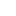喜欢 | 1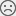不喜欢 | 0

JAVA工程师交流群 +加入

WEB/H5前端交流群 +加入•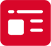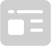索取资料
•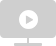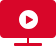答疑解惑
•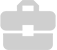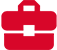技术交流
•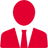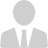职业测评
•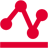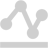面试技巧
•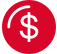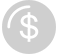高薪秘笈
JAVA30天热搜词 更多>>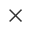Java全栈开发
WEB前端+H5沪公网安备 31011502005948号    ICP许可  沪B2-20190160